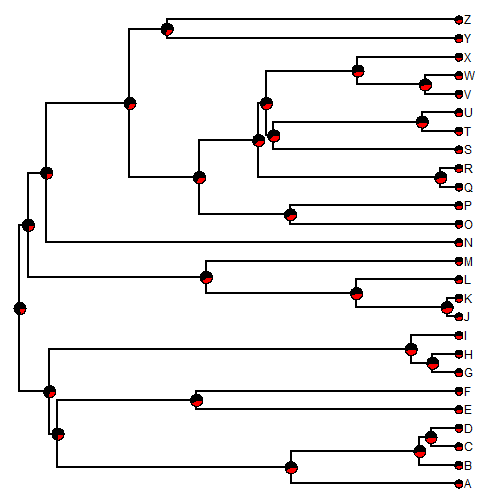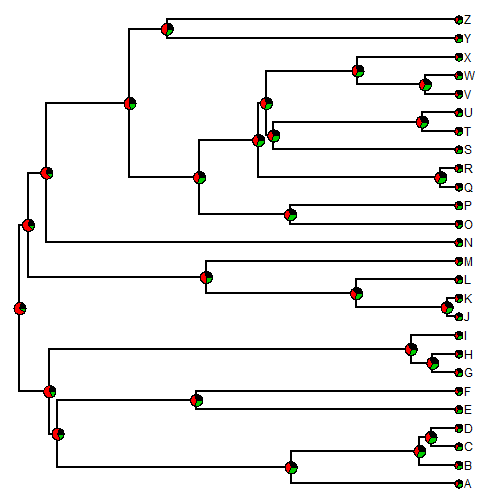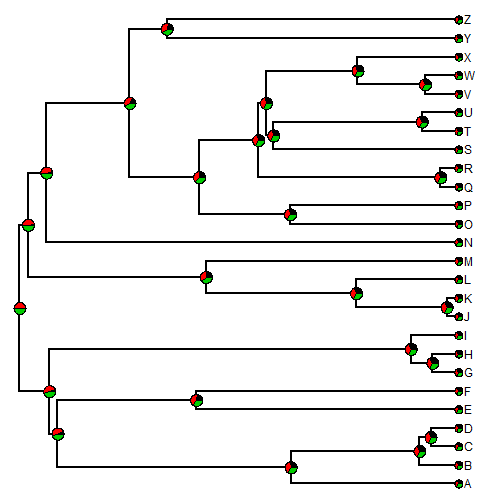## Wednesday, May 11, 2016

### New ways to set the ancestral state in `sim.history`

I just updated `sim.history`, a function which conducts simulations up a phylogeny using the Mk model. This function simulates the complete character history, producing an object of class `"simmap"` (or `"multiSimmap"` for multiple simulations).

The updates firstly fix a bug in how ancestral states were being set for `nsim > 1`, but also added the following features:

1) If `anc` is supplied as a character vector, then the ancestral state will be picked randomly from the vector.

2) If `anc` is supplied as a numeric vector with names, then the ancestral state will be picked in proportion to the values in the vector. (Hopefully these are probabilities, but if they are not they should be rescaled internally. Obviously, they must be positive.

Here is a demo to show that it works more or less as you'd expect:

``````library(phytools)

tree<-pbtree(n=26,tip.label=LETTERS,scale=1)
Q<-matrix(c(-1,1,1,-1),2,2)
rownames(Q)<-colnames(Q)<-letters[1:2]
Q
``````
``````##    a  b
## a -1  1
## b  1 -1
``````
``````## first for two states with unequal probabilities
trees<-sim.history(tree,Q,anc=setNames(c(0.75,0.25),letters[1:2]),
nsim=1000)
``````
``````## Done simulation(s).
``````
``````trees
``````
``````## 1000 phylogenetic trees with mapped discrete characters
``````
``````obj<-summary(trees)
obj
``````
``````## 1000 trees with a mapped discrete character with states:
##  a, b
##
## trees have 12.096 changes between states on average
##
## changes are of the following types:
##      a,b   b,a
## x->y 7.1 4.996
##
## mean total time spent in each state is:
##              a         b    total
## raw  7.9030266 4.1353292 12.03836
## prop 0.6564872 0.3435128  1.00000
``````
``````## state frequencies at root:
obj\$ace[1,]
``````
``````##     a     b
## 0.755 0.245
``````
``````plot(obj)
````````````## next for a vector of possible values
trees<-sim.history(tree,Q,anc=c("a","b"),nsim=1000)
``````
``````## Done simulation(s).
``````
``````trees
``````
``````## 1000 phylogenetic trees with mapped discrete characters
``````
``````obj<-summary(trees)
obj\$ace[1,]
``````
``````##     a     b
## 0.503 0.497
``````
``````## nothing
trees<-sim.history(tree,Q,nsim=1000)
``````
``````## Done simulation(s).
``````
``````trees
``````
``````## 1000 phylogenetic trees with mapped discrete characters
``````
``````obj<-summary(trees)
obj\$ace[1,]
``````
``````##     a     b
## 0.495 0.505
``````
``````## now three states
Q<-matrix(c(-2,1,1,1,-2,1,1,1,-2),3,3)
rownames(Q)<-colnames(Q)<-letters[1:3]
Q
``````
``````##    a  b  c
## a -2  1  1
## b  1 -2  1
## c  1  1 -2
``````
``````## simulate with unequal probabilities
trees<-sim.history(tree,Q,anc=setNames(c(0.2,0.7,0.1),letters[1:3]),
nsim=1000)
``````
``````## Done simulation(s).
``````
``````trees
``````
``````## 1000 phylogenetic trees with mapped discrete characters
``````
``````obj<-summary(trees)
obj\$ace[1,]
``````
``````##     a     b     c
## 0.199 0.678 0.123
``````
``````plot(obj)
````````````## draw from a vector
trees<-sim.history(tree,Q,anc=c("b","c"),nsim=1000)
``````
``````## Done simulation(s).
``````
``````obj<-summary(trees)
plot(obj)
````````````obj\$ace[1,]
``````
``````##   a   b   c
## 0.0 0.5 0.5
``````

That's all for now. This update can be installed from GitHub using devtools as follows:

``````library(devtools)
install_github("liamrevell/phytools")
``````